×
Log in to StudySoup
Get Full Access to WSU - MECHA 850 - Class Notes - Week 2
Join StudySoup for FREE
Get Full Access to WSU - MECHA 850 - Class Notes - Week 2

Already have an account? Login here
×
Reset your password

WSU / OTHER / Mechanical Engineering 850 / wsu mechanical engineering

# wsu mechanical engineering Description

##### Uploaded: 02/21/2017
68 Pages 129 Views 0 Unlocks
Reviews

Electrochemistry of Corrosion http://www.youtube.com/watch?v=XMr4vse7Ybo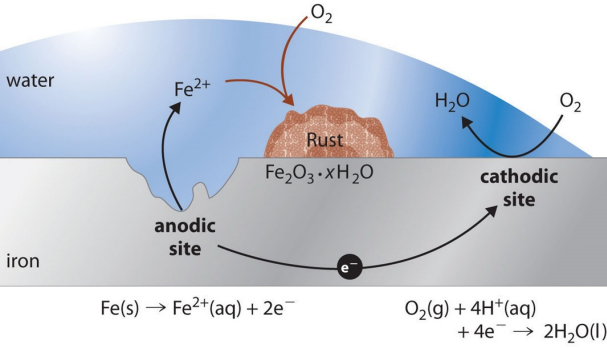http://www.youtube.com/watch?feature=endscreen&v=QYd9ENn1nP0&NR=1 https://www.youtube.com/watch?v=_gBaOH4X9CQCorrosion process of metals can be influenced by many factors.  Electrochemistry Electrochemistry is a branch of chemistry that studies chemical reactions  which take place in a solution at the interface of an electron conductor (a metal  or a semiconductor) and an ionic conductor (the electrolyte), which involves  electron transfer between the electrode and the electrolyte or species in the  solution.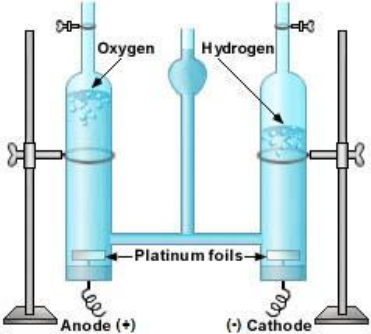Reduction of a metal with its  environment is called corrosion, so it  is an electrochemical process. It may  occur in an aqueous media (water  and dissolved ions) or high  temperature (oxygen and other  gases). We have anode and cathode in an electrochemical cell. Water can be converted to its component elemental gasses, H2 and O2 through  the application of an external voltage. It is an electrochemical process, too.Definitions of Anode The electrode at which chemical reaction (oxidation or anodic reactions) occur  is called anode. For corrosion, these typically correspond to the various metal  dissolution reactions, such as:  Zn → Zn2+ + 2e- Zinc corrosion (Zn metal ionizes, giving 2e-) Fe → Fe2+ + 2e- Iron corrosion Al → Al3+ + 3e- Aluminium corrosion Fe2+ → Fe3+ + e- Ferrous ion oxidation H2 → 2H+ + 2e- Hydrogen oxidation 2H2O → O2 + 4H+ + 4e- Oxygen evolutionIn other words, the anode is an electrode at which currents leaves to return to  the electrolyte. It continuously produces electrons when the reaction starts.  Definitions of Cathode Reduction or cathodic reactions result in the consumption of electrons, and for  corrosion these typically correspond to the oxygen reduction or hydrogen  evolution reactions. In other words, the cathode is an electrode at which  currents enters from the electrolyte. O2 + 2H2O + 4e- → 4OH- Oxygen reduction 2H2O + 2e- → H2 + 2OH- Hydrogen evolution Cu2+ + 2e- → Cu Copper plating Fe3+ + e- → Fe2+ Ferric ion reduction Zn → Zn2+ + 2e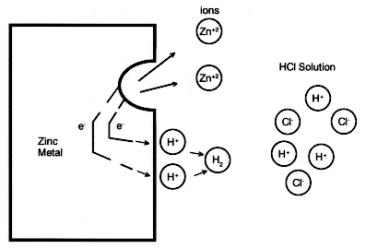2H+ + 2e- → H2 (g) Zn + 2H+ → Zn2+ + H2(g) Metal Corrosion       The electrochemical cell set up between anodic and cathodic sites on an iron    surface simultaneously undergoing corrosion.                  Fe3+(aq) + 3 OH−(aq) ⮀ Fe(OH)3(s) (rust-color)     Ni2+(aq) + 2 OH−(aq) ⮀ Ni(OH)2(s) (green)      Mn2+(aq) + 2 OH−(aq) ⮀ Mn(OH)2(s) (light brown) Al3+(aq) + 3 OH−(aq) ⮀ Al(OH)3(s) (white, gelatinous)  Zn2+(aq) + 2 OH−(aq) ⮀ Zn(OH)2(s) (white)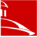The same metallic surface exposed to an aqueous electrolyte usually  possesses sites for an oxidation (or anodic chemical reaction) that produces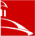electrons in the metal, and a reduction (or cahtodic reaction) that consumes the  electrons produced by the anodic reaction. Electrochemical Half-Cell An electrochemical cell is composed to two compartments or half-cells, each  composed of an electrode dipped in a solution of electrolyte. These half-cells  are designed to contain the oxidation half-reaction and reduction half-reaction  separately as is shown below. During the oxidation of zinc, the zinc electrode will  slowly dissolve to produce zinc ions (Zn2+), which enter  into the solution containing Zn2+(aq) and SO42-(aq) ions.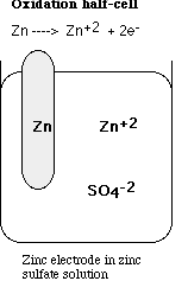The half-cell, called now the anode, is the site at which the oxidation of zinc  occurs as is shown below:  Zn(s) ----------> Zn2+(aq) + 2e Electrochemical Half-Cell The half-cell, called now the cathode, is the site at which reduction of copper occurs as shown below. Cu2+(aq) + 2e- -------> Cu(s)  When the reduction of copper ions (Cu2+) occurs,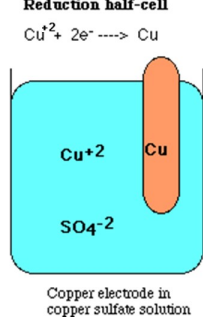copper atoms accumulate on the surface of the solid  copper electrode – called electroplating or metallic  coating.  This coating can passivate the surface to increase the  corrosion resistance of the metal surfaces. Electrochemical (Galvanic) Cells The two half-cells are connected externally to make galvanic cell. In this  arrangement, electrons provided by the oxidation reaction are forced to travel  via an external circuit to the site of the reduction reaction. The fact that the  reaction occurs spontaneously once these half cells are connected indicates  that there is a difference in potential energy.This difference in potential energy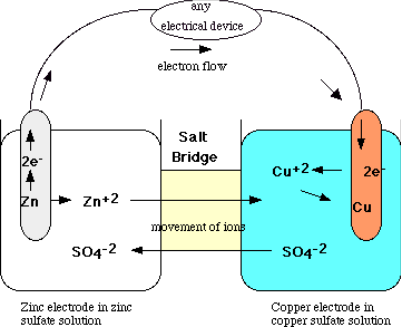is called an electomotive force (emf) and is measured in terms of  Volts. The zinc/copper cell has an  emf of about 1.1 Volts under  standard conditions.Pollution by Corrosion Acid mine waste pollution is caused by the physical and chemical weathering of  a very common mineral (pyrite – iron II sulfide). 4FeS2(s) + 14O2(g) + 4H2O(l) ---> 4Fe2+(aq) + 8SO42-(aq) + 8H+(aq)  Iron II ions and acidic hydrogen ions are released into the waters that runoff  from the mine drainage tunnels or tailings piles. Iron II ions are oxidized to form  iron III ions as shown in the following reaction:  4Fe 2+(aq) + O2(g) + 4H+(aq) ---> 4Fe3+(aq) + 2H2O(l)  4Fe3+(aq) + 12H2O(l) ---> 4Fe(OH)3(s) + 12H+(aq) The iron III ions now hydrolyze in water to form  iron III hydroxide as is seen above, which may  cause environmental pollution.  Sulfuric acid can also form during this process  to reduce the pH level of the liquid. The Standard emf Series This table represents the  corrosion tendency for  several metals; thus, at  the top, gold is noble, or  chemically inert.  At the bottom, the metals  become increasingly  more active, that is, more  susceptible to oxidation.  Li has the highest  reactivity to be used in  Li-ion batteries. Galvanic Series • Ranking of the reactivity of metals/alloys in seawater c idohtac eromc idona erom) treni() evitca(Platinum Gold Graphite Titanium Silver 316 Stainless Steel (passive) Nickel (passive) Copper Nickel (active) Tin Lead 316 Stainless Steel (active) Iron/Steel Aluminum Alloys Cadmium Zinc Magnesium The galvanic series (or  electropotential series)  determines the nobility of metals  When two metals are submerged  in an electrolyte, while electrically  connected, the less noble (base)  will experience galvanic  corrosion.  Galvanic reaction is the principle  upon which batteries are based.Table 17.2, Callister & Rethwisch 9e. Source is M.G. Fontana, Corrosion Engineering, 3rd ed., McGraw Hill Book Company, 1986. Reprinted with permission) Source of Electricity The redox reaction (combination of anodic and cathodic reactions) in a galvanic  cell is a spontaneous reaction. For this reason, galvanic cells are commonly  used as batteries. Galvanic cell reactions supply energy which is used to  perform work. The energy is harnessed by situating the oxidation and reduction  reactions in separate containers, joined by an apparatus that allows electrons to  flow. A common galvanic cell is the Daniell cell, shown below.  Corrosion is a destructive process, but the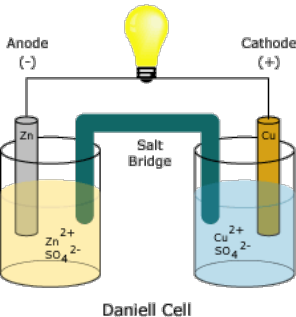reactions can produce enough electricity for  useful applications, such as cell phones,  laptops, videos, cameras, music players, etc. Metal Ion Hydrolysis Hydrolysis is a chemical decomposition process that uses water to split  chemical bonds of substances. Here, Al metal ions react with water (a  hydrolysis reaction): Al3+ + 3H2O → Al(OH)3 + 3H+ (atomic and charge balance) 2Al3+ + 3H2O → Al2O3 + 6H+ Note that in an electrochemical reaction, we have the same number of each  atom on each side of the equation, and the same overall charge.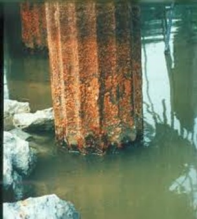Hydrolysis of iron beam in waterGibbs Free Energy Change  ∆ =− G nFE Gibbs free energy  where ∆G is Gibbs free energy, E is standard potential of the reaction (Volt), n is number of electrons involved in the chemical reaction, and F is the Faraday  constant (96,500 C/eq) (eq = equivalent).  Ex: How do you determine the Faraday constant?F eN =A F = 96,500 C/mol e ≈ 1.6021766×10−19 C NA ≈ 6.022141×1023 mol−1 When the concentrations of the solutions  are the same, the cell potential Ecell will be:  o o EE E cell cathode anode = − Nernst equation given below is used to calculate the cell potential Ecell when the concentrations are not the same in the solutions;  0.059 ( ) o o E E E cell cathode anode Log Q 1 Cal. = 4.184 Joules = −− n 10 where Ecell is cell electrode potential, Eo is standard potential and Q is the ratio  of molar ion concentrations. Zn-Cu Galvanic Cell Potential oo o EE E cell cathode anode = − 0.34 ( 0.76) 1.10 V o Ecell= −− =A galvanic cell can be constructed by inserting a copper strip into a beaker that  contains an aqueous 1 M solution of Cu2+ ions and a zinc strip into a different  beaker that contains an aqueous 1 M solution of Zn2+ ions. The two metal strips  are connected by a wire that allows electricity to flow, and the beakers are  connected by a salt bridge. Later, as is seen Zn anode corrodes significantly.  Free Energy Changes of Hydrolysis Tendency for any chemical reaction to go is measured by Gibbs Free Energy Change ∆G.  ∆ =− G nFEMg + H2O(l) + ½O2(g) ------???? Mg(OH)2(s) ∆G° = -142,600 Cal. Cu + H2O(l) + ½O2(g) ------???? Cu(OH)2(s) ∆G° = -28,600 Cal. Au + 3/2H2O(l) + 3/2O2(g) ------???? Au(OH)3(s) ∆G° = +15700 Cal. Large negative ∆G indicates the higher tendency to react with water and  oxygen for corrosion/oxidation. Thermodynamically, at normal conditions gold does not corrode in aqueous  media to form Au(OH)3 (impossible). ∆G value for gold is positive.  Pourbaix Diagram In chemistry, a Pourbaix diagram, also known as a potential/pH diagram  (pE/pH), maps out possible stable (equilibrium) phases of an aqueous  electrochemical system. Predominant ion boundaries are represented by lines.  As such a Pourbaix diagram can be read much like a standard phase diagram  with a different set of axes. Similarly to phase diagrams, they do not allow for  reaction rate or kinetic effects.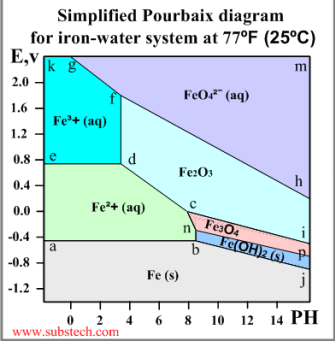• a-b: Fe(s) = Fe2+(aq) + 2e- Redox reaction  independent of PH. The equilibrium occurs at  the electrode potential value -0.44V, which is  equal to the standard electrode potential of iron • e-d: Fe2+(aq) = Fe3+(aq) + e- Redox reaction  independent of PH. • d-f: 2Fe3+(aq) + 3O2- = Fe2O3(s) Non-redox  reaction dependent on PH. • b-n: Fe2+(aq) + 2OH-(aq) = Fe(OH)2(s) Non redox reaction dependent on PH. • c-d: 2Fe2+(aq) + 3H2O = Fe2O3(s) + 6H+(aq)  +2e- Redox reaction dependent on PH. • b-j: Fe(s) + 2OH-(aq) = Fe(OH)2(s) + 2e Redox reaction dependent on PH. https://www.youtube.com/watch?v=3PDqGO39F8o1 1 Pourbaix Diagrams (I) 3 3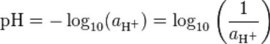1 2 1) Corrosive regions: the most stable form is the metal cation or any non  protective anion corrosion will occur until the metal is consumed (possible). 2) Immune region: corrosion is thermodynamically impossible. 3) Passive regions: an insoluble protective layer (hydroxide or oxide layer) is  the most stable form; initial corrosion will occur until a protective layer is formed Pourbaix Diagrams (II) Pourbaix Diagrams explains a compact summary of thermodynamic data in    the form of potential – pH diagrams, which relate to the electrochemical and  corrosion behavior of any pure metal (not alloy) in water.  1.21.2 0.80.8 0.40.4 l l aiaittnneettooPP0.00.0 --0.40.4 --0.80.8 --1.21.2 --1.61.6 --2.02.0 Al3+Al2O3AlO2- Al --2.42.4 00 77 1414 Potential – pH diagram for water Potential – pH diagram for Al metalEquilibrium Phases Which of the states are stable and which of the states are unstable to corrode in  the diagrams below? Potential-pH diagram for Cu-water at  25 C, 10-6 M Cu.  Pourbaix diagram for nickel with a  concentration of 10-6 mol/L  corresponding to a concentration of 58  µg/L nickel.Reference Electrodes The purpose of the reference electrode is to complete the measuring circuit and  provide a stable and reproducible potential against which the indicator  electrode is compared. Usually, a salt bridge is formed from the sample through  the electrolyte to the silver-silver chloride reference element.1. Standard Hydrogen Electrode (SHE)  2. Silver/Silver chloride 3. Calomel (mercury/mercurous chloride) 4. Mercury/Mercurous Sulfate 5. Copper Sulfate   Electrode Names Equilibrium Reactions Hydrogen 2 H+ + 2 e-= H2 Silver chloride AgCl + e-= Ag + Cl  Calomel Hg2Cl2 + 2 e-= 2 Hg + 2 Cl  Mercurous sulfate Hg2SO4 + 2 e-= 2 Hg + SO42- Mercuric HgO + 2 e-+ 2 H+ = Hg + H2O Copper sulfate Cu2+ + 2 e-= Cu (sulfate solution)

## Ex: How do you determine the Faraday constant?If you want to learn more check out asu bus tracker

Standard Hydrogen Electrode (SHE) Working principles: 1. Platinized platinum electrode  2. Hydrogen blow  3. Solution of the acid with activity of H+ = 1 mol/L 4. Hydroseal for prevention of the oxygen  interference  5. Reservoir through which the second half element of the galvanic cell should be  attached. This creates an ionically conductive  path to the working electrode of interest. Standard Calomel ElectrodeSilver/Silver ChlorideArrhenius Equation Chemical intuition suggests that the higher the temperature, the faster a given  chemical reaction will proceed. Quantitatively this relationship between the rate  a reaction proceeds and its temperature is determined by the Arrhenius  Equation. At higher temperatures, the probability that two molecules will  react/collide is higher. This higher reaction/collision rate results in a higher  kinetic energy, which has an effect on the activation energy of the reaction. The  activation energy is the amount of energy required to ensure that a reaction  takes place.  EA Y = 1.342X + 2.012 R2 = 0.985RT k A − = exp where k is the rate coefficient  constant, A is a pre-exponential  factor constant, EA is the  activation energy, R is the  universal gas constant (8.31  J/K.mol), and T is the temperature  (in Kelvin). Equilibrium Constant The equilibrium constant (K) is a quantity characterizing a chemical  equilibrium in a chemical reaction, which is a useful tool to determine the  convertion of various reactants or products in a system where chemical  equilibrium occurs. aA + bB → cC + dD  d D c C K = [ ] [ ] b B a A [ ] [ ] Although chemical equilibrium is defined kinetically (forward and backward  rates are equal), its properties can be studied thermodynamically, i.e., from the  Gibbs free energies of the reactants and products:  ∆G = −RT ln K where R is the universal gas constant (8.31  J/K.mol), and T is the temperature (in Kelvin)Corrosion Rate The corrosion rate, or rate of metal removal as a result of the chemical reaction,  is an important corrosion parameter. This may be expressed as the corrosion  penetration rate (CPR), or the thickness loss of material per unit time:  KW CPRρ =At mm/year or mil/year (mpy) W = Weight loss t = Exposure time ρ = Density of the specimen A = Specimen area K = Constant (87.6 for mm/year and 534 for mil/year )  For most application, the corrosion rate is less than 0.50 mm/year. • 1 mil is 25.4 µm or 0.001 in. Example Problem 1 Calculate the value of the equilibrium rate constant K for the reaction if 0.1908  moles of CO2 and 0.0908 moles of H2 react with to form 0.0092 moles of CO,  and 0.0092 moles of H2O vapor in a 1.00 L reaction vessel.  Solution: First of all, let’s write the chemical reaction; CO2(g) + H2(g) → CO(g) and H2O(g) ] 2 [ ][ CO H O K = ] 2 ][ 2 [ CO H Substitute each concentration into the equilibrium expression and calculate the  value of the equilibrium constant.  [0.0092][0.0092] K = [0.1908][0.0908] 3 K x 4.9 10− =The value of the equilibrium constant for the  reaction above.Example Problem 2 A one-liter vessel contains 1.60 moles ammonia NH3 after the reaction of 0.80  moles N2, and 1.20 moles of H2. What is the equilibrium rate constant K of the  reaction given below?  N2(g) + 3H2(g) <--> 2NH3(g)  Solution: The equilibrium rate constant K of the reaction can be calculated  below: 2]3 [ NH K = 3] 2 ][ 2 [ N H 2 [1.60] K = 3 [0.80][1.20] K = 1.85Example Problem 3 If the Gibbs free energy ∆G values of the reaction are 60890 J/mol and -165420  J/mol at the temperatures T of 300 K and 1200 K, respectively, calculate the  equilibrium rate constants K of the reaction at 300 K and 1200 K temperatures.  The value of the gas constant R is 8.31 J/mol.K.  ZnO(s) + C(s) -----> Zn(s) + CO(g)  Solution: Using the equation below, one can calculate the equilibrium rate  constants Kp at 300 K and 1200 K:  o o G KRT G log K RT ∆ =− G RT K ln ln p = − ∆ p = − ∆ 10 2.303 At 300 K, the equilibrium rate constant:  60890 = −10 10.60 p log K = −11 2.512 10 K x p− = 2.303 8.314 300 p log Kx x 10 At 1200 K, the equilibrium rate constant:  − = −10 7.197 p log K =7 1.574 10 K x p= 165420 2.303 8.314 1200 p log Kx x 10 The calculated rate constants indicate that the chemical reaction at 1200 K will  be much faster (more than 17 orders of magnitudes) compared to 300 K. Example Problem 4 A chemical reaction, 2NO2(g) -----> 2NO(g) + O2(g), has a rate coefficient k of  1.0x10-10 /sec at 27 °C (300 K). What is the activation energy EA of the reaction  at this temperature? The values of the gas constant R and the pre-exponential  factor constant A are 8.31 J/K.mol and 2.1x10+9 / sec, respectively.  Solution: Use the Arrhenius equation to solve this problem. Constant A and the  activation energy EA do not vary much with temperature. 2NO2(g) -----> 2NO(g) + O2(g)  EA RT k A − = exp x x − − = EA 10 9 (8.31)(300) 1.0 10 2.1 10 exp EA = 111 kJ/mol The activation energy of the reaction at 27 °C.Example Problem 5 After the analysis of a submerged ship, a piece of corroded metal plate was  found near the ship. It was estimated that the original area of the plate was 900  cm2 and that approximately 12.8 kg had corroded away during the submersion.  Assuming a corrosion penetration rate of 5 mm/yr for this metal in a seawater,  estimate the time of the submersion in years. The density of the metal is 4.67  g/cm3.  Solution: Using values for the various parameters given in the problem  statement yields the following value for t: =Corrosion penetration rate (CPR) parameters include, constant  KW CPRρ At K (87.6), weight loss W, density ρ, exposed area A, and  exposure time t. ( ) 6 (87.6) 12.8 kg)(10 mg/kg  = 4.67 g/cm 900 cm (5 mm/yr) t ( )( ) 3 2 4 t= 5.34 10 hrs = 6.09 yrs ⋅ The exposure time of  the metal plateReferences 1. Revie, R.W. and Uhlig, H.H. “Corrosion and Corrosion Control –An  Introduction to Corrosion Science and Engineering, 4rd Edition,” Wiley Interscience, 2008. 2. Roberge, P. R. “Corrosion Engineering Principles and Practice,” McGraw  Hill, 2008. 3. Ahmad, Z. “Principles of corrosion engineering and corrosion control,”  IChemE, 2006. 4. K.R. Trethewey and J. Chamberlain, “Corrosion for Students of Science and  Engineering,” Longman Scientific & Technical, 1992.  5. Callister, W.D. “Materials Science and Engineering An Introduction – 7th  Edition,” Wiley, 2007 6. http://chemistry.about.com/library/weekly/aa082003a. 7. htmhttp://electrochem.cwru.edu/ed/encycl/art-c02-corrosion.htm 8. http://www.cp.umist.ac.uk/lecturenotes/Echem/Electricityfile.html 9. http://chem.ch.huji.ac.il/~eugeniik/refelectrodes.htm 10.http://www.sci-journal.org/reports/vol5no1/a3/tc2.htm 11.http://www.corrosion-doctors.org/ 12.http://www.ntu.edu.sg/home/asjqiu/me/ME303L01.HTMBasic Principles of Metallic  Corrosion https://www.youtube.com/watch?v=DsHNrHccadE https://www.youtube.com/watch?v=emdmDXKsXYI https://www.youtube.com/watch?v=CnFuMWpmJzgThe potential difference across a cell can be calculated using the Nernst  equation at different solution concentrations.  Electrochemical Basics • Corrosion is an electrochemical phenomena – The simultaneous combination of electrical & chemical processes • Techniques involve either or both of: – Measuring voltage difference (thermodynamic) – Measuring current flow (kinetic) • Working electrode – Equipment material • Reference electrode – Maintains constant potential • Even at large currents • Counter electrode – Allows infinite current GAMRY corrosion cellDriving Force • A driving force is necessary for electrons to flow between the  anodes and the cathodes. • The driving force is the difference in potential between the  anodic and cathodic sites/reactions.  • This difference exists because each oxidation or reduction  reaction has associated with it a potential determined by the  tendency for the reaction to take place spontaneously. The  potential is a measure of this tendency. Mixed Potential Mixed potential is the effective potential of the metal surface in contact with an  electrolyte that is driving electrochemical corrosion. Mixed potential theory  explains metal corrosion as a reaction of two or more electrodes working  simultaneously at the interface of the given metal surface and electrolyte. It  needs to be controlled for the elimination of the corrosive reaction. By  cathodically polarizing the cathode, the corrosion current density can be  reduced to eliminate corrosion. Mixed potential occurs at zero net current of  anodic and cathodic reactions (anodic current = cathodic current; ia = ic).  Mixed potential theory is used to predict the corrosion rates of metals.  The study of mixed potential is important because: • It helps explain cathodic protection, which is an effective method of protecting  buried pipelines, storage tanks, structures and marine equipment • It is useful for fine tuning and calibration of measuring as well as monitoring  devices used for corrosion protection systems, and useful for different  environmental conditions • It is useful in the design of corrosion protection systems and the selection of  required components    N2 +O2 Mixed Potential Theory • All reactions occur at the metal surface e M     0 H2O O2 OH Mm+ O2 + 2H2O + 4e???? 4OH M ???? M m+ + me i • Potential is estimated to be the same  on all the surface of the metal • At the final potential, oxidation rate =  reduction rate • When oxidation rate is equal to the  reduction rate, steady state potential  occurs, called corrosion potential (or    mixed potential) V = + β l aitnetoPanodic E a i 0 log o i V E anodic a io = + β Anodic potential  E’ log io at which βa is the slop, ia is current  density and iO is exchange current  density. log o i V E cathodic a io = − β Cathodic potential log i Basic Principles of Metallic Corrosion: Mixed  Potential Tacking into account M = Mm+ + me and O2 + 2H2O + 4e = 4OH- (aerated  neutral solution).  ????   EE’O2,OH E’M,Mm+ log i ???? According to the mixed-potential theory, any electrochemical reaction can be  algebraically divided into separate oxidation and reduction reactions with no net  accumulation of electrical charge/current.Basic Principles of Metallic Corrosion:  Mixed Potential (Ecorr, icorr) are potential and ????   EE’O2,OH ECORR E’M,Mm+ current values, respectively, when a metal is in an aqueous solution (in this case an aerated neutral solution) https://www.youtube.com/watch?v=iZEAkiGrPTwlog iCORR log i ???? Mixed Potential Diagram Zn = Zn2+ + 2e- Anodic reaction 2H+ + 2e- = H2 Cathodic reaction Polarization of anodic and  cathodic half-cell reactions  for zinc in acid solution to  give a mixed potential, Ecorr,  and a corrosion rate (current  density), icorr. Each has its own half-cell  electrode potential and  exchange current density.  The two half-cell electrode  potentials cannot coexist  separately on the same  electrically conductive zinc  surface. The graphical form is called mixed potential, polarization or Evans  diagrams. Tafel Region Extrapolation Corrosion current (Icorr) is  the current between the  anodic and cathodic sites,  and normally both the  anodic metal dissolution  process and the supporting  cathodic process occur at  microscopically adjacent  points on the metal  surface.  Curve fitting over mix region can be used to determine Ecorr and Icorr, the free  corrosion potential and the corrosion current. This will provide the CPR for the  metals in the solutions. Tafel Curve of Iron At the corrosion potential, the  rate of hydrogen evolution is  equal to the rate of metal  dissolution, and mixed point  corresponds to the corrosion  rate of the system in terms of  current density. The sum of  anodic oxidation currents  must equal the sum of  cathodic reduction currents.Mixed potential diagram of iron with the  aerated neutral solution at 25°C. Tafel constants  must be calculated from both the anodic and  cathodic portions of the Tafel plot. Gamry  software can find the Tafel corves for each  material and fits the curves with the test results.  Electrical Double Layer (I) The electrical double layer describes the  variation of electric potential near a surface,  and has a large bearing on the behavior of  surfaces in contact with the solution.Electrical Double Layer (II) Development of a net charge at the  particle surface affects the  distribution of ions in the  surrounding interfacial region,  resulting in an increased  concentration of counter ions (ions  of opposite charge to that of the  particle) close to the surface. Thus,  an electrical double layer exists  round each particle. Zeta potential is electrical potential  in the interfacial double layer at the  location of the slipping plane versus  a point in the bulk fluid away from  the interface. Zeta Potential and pH Point of zero chargeZeta potential of the surfaces can be changed by changing the pH values (e.g.,  using HCl and NaOH solutions). The Iso-electric point (point of zero charge) is  a pH value at which the zeta potential is nearly zero. Zeta potential can affect  the polarization and corrosion rates of the surfaces.  Electrochemical Impedance  Spectroscopy (EIS) - EIS has been successfully applied to the study of corrosion systems and been proven to be a powerful method for measuring corrosion rates. - To access the charge transfer resistance or polarization resistance that is proportional to the corrosion rate at the monitored interface, EIS results have to be interpreted with the help of a model of the interface. - An important advantage of EIS over other techniques is the possibility of using very small amplitude signals without significantly disturbing the properties being measured. To make an EIS measurement, a small amplitude signal, usually a voltage between 5 to 50 mV, is applied to a specimen over a range of frequencies of 0.001 Hz to 100,000 Hz. - The EIS instrument records the real (resistance) and imaginary (capacitance) components of the impedance response of the system.Coating Damages Photograph of (a) epoxy  coated (b) polyaniline (PANI)  coated (c) hydrophobic  polyaniline-SiO2 composite  (HPSC) coated, and (d)  HPSC + inhibitor for mild  steel specimens after 35  days of exposure to salt  spray test.  The coating films were  purposely cut (X mark) to  determine the coating  behaviors against corrosion  attacks. Coating Resistance and Capacitance Equivalent circuit for a perfect coating with  resistance R and capacitance C.  Equivalent circuit for a damaged coating Cc is the capacitance of the coating Cdl is a double layer capacitance  Rs is solution resistance Rct is charge transfer resistance Rpo is the pore resistance of coating  Nyquist plot for an  perfect coatingNyquist plot for a  damaged coating http://www.gamry.com/application-notes/basics-of-electrochemical-impedance-spectroscopy/ Series Connection of R-C Circuit Series connection of the Randle’s circuit or Randle’s cell (R-C) model for real  (ZRe) and imaginary (ZIm) resistance / impedance. The impedance at any frequency w has a complex imaginary number j.Z Z R ZC R jwC R 1 ( ) 1 = + = + = − − ZRe = R , ZIm = -1/(wC) 2 * 2 | | Z Z Z = R + X C = ⋅ Xc1 j wC = Xc : capacitive reactance -ZIm Xc wC Z(w) w : angular frequency High frequency : Z = R Low frequency : Z = ∞ tanθ = ZIm/ZRe = Xc/R = 1/ωRC θ R ZRe 2 2 2 2 Re | | C Z = Z + Z = R + X Im Parallel Connection of R-C Circuit Parallel connection of the R-C circuit = + = + = + −1 1 1 1 1 1 jwC Z ZR ZC R jwC R 1 ( ) 1 − 1 R jwC R   wR C 2 = +  = + − + Nyquist plot Z 2 2 2 j 1 1  R Z+ w R C 2 2 2 2 w R C wR C Z+− = =2 2 2 , Re 2 2 2 1 w R C Im 1 w R C High frequency : Z = 0 Low frequency : Z = R Full Randle’s Circuit Warburg Impedance, W  - Diffusion control reaction - Usually observed in low frequency region Warburg diffusion element is a  common diffusion circuit element  that can be used to model semi W −1/ 2 −1/ 2 =σω − jσω High freq. : W = 0 Low freq. : W = ∞ infinite linear diffusion process.   σ RT 1 1 m ho / ''Z20K 18K 15K 13K 10K 8K 5K 3K 0 Nyquist plot ← w slope=1

## What is the equilibrium rate constant K of the reaction given below?We also discuss several other topics like ucsc economics courses
We also discuss several other topics like What was the growth rate in RGDP in 2012?
We also discuss several other topics like ecn 211

0 3K 5K 8K 10K 13K 15K 18K 20K Z' / ohm Rs Rs+Rct= + 2 2 1/ 2 1/ 2 ( ) n F 2 Cox Dox Cred Dred ( ) σ : Warburg impedance coefficient R : ideal gas constant T : absolute temperature n : the number of electron transferred F : Faraday’s constant Cox : conc. of oxidation species Cred : conc. of reduction species Dox : diffusivity of oxidation species Dred : diffusivity of reduction species Full Randle’s Circuit Randle’s circuit is one of the simplest and  most common cell models. It includes a  solution resistance, a double layer capacitor  and a charge transfer or polarization  resistance. In addition, the Randle’s cell  model is often the starting point for other  more complex models.5 Example for the R-C circuit  60 m ho / ''Z20K 18K 15K 13K 10K 8K 5K 3K 0 Nyquist plot ← w

## What is the activation energy EA of the reaction at this temperature?If you want to learn more check out reggienet isu
We also discuss several other topics like engr 151

4 4 ) o(4 )Z(gol4 3 3 Bode plot

50 40 30 20 10 0 phase / deg(+) 0 3K 5K 8K 10K 13K 15K 18K 20K Z' / ohm -1 0 1 2 3 4 log(f) Equivalent Circuit of Coating Porous Coating Damaged Coating This Nyquist plot is generated assuming  that solution resistance Rs = 20 Ω, and  Nyquist plot Rs Rp polarization resistance Rp = 250 Ω. Diameter of the semicircle is equal to the  polarization resistance (in this case 250Ω). Corrosion Cell Potentials Overall cell potential at standard condition (1 M): ( ) oanode E ocathode cell E o E = − Nernst equation (not standard condition of solution concentrations):  [ ] ( ) ln++ E ocathode o RT anode n M = − − n E cell E anode nF [ ] cathode M where F is the Faraday constant (96,500 C/eq. or 23060 Cal/V-gram eq.), R is  the gas constant (8.314 Joules/Kelvin or 1.985 Calories/C), T is absolute  temperature in K, n is number of electrons, and M are the anode and cathode  molar ion concatenations. At temperature 25 °C (298 K), Nernst equation will  be:  E ocathode o 0.0592 anode n M + [ ] log = − − n ( )+ E cell E anode n cathode M [ ] Example: When temperature is 298 K, 2.303xRxT/F constant value in the  formula will be 0.0592. Demonstration is given below: RTx x (8.31 J/mol-K)(298 K) ln( ) = (2.303) log ( ) ( )(96,500 C/mol) nF n 0.0592 = log ( ) x nExample Problem 1 One-half of an electrochemical cell consists of a pure nickel electrode in a  solution of Ni2+ ions, and the other half is a cadmium electrode immersed in  a Cd2+ solution. Faraday constant is 23060 Cal/Volt.  a) If the cell is a standard one, write the spontaneous overall reaction and  calculate the voltage that is generated.  b) Calculate the cell potential at 25 °C if the Cd2+ and Ni2+ concentrations are  0.5 and 10-3 M (not standard), respectively.  c) What is the Gibbs free energy change of the system in the later conditions  and what does that mean? Table provides standard  electrode potentials of  some metals. Select the  potential values from the  table.  Noble metals don’t  corrode easily, but the  base metals. Answer 1a a) The cadmium electrode will be oxidized and the nickel reduced because  cadmium has lower electrode potential (-0.40 V) than nickel (-0.23 V) in the emf  series; therefore, spontaneous reaction will be:  Cd → Cd2+ + 2e- V = -0.40 V (anode) Ni2+ + 2e- → Ni V = -0.23 V (cathode) Ni2+ + Cd → Ni + Cd2+  E ocathode cell E o E = − ( ) oanode cell V o E = (−0.23 − (−0.40)) = +0.17 Voltage generated  during this reaction Answer 1bb) Based on the information given above, Nernst equation may be used to solve  the problem because the half-cell solution concentrations are no longer 1 M. Ni2+ + Cd → Ni + Cd2+ Overall chemical reaction anode n M E ocathode o 0.0592 + [ ] log = − − n ( )+ E cell E anode n [ ] cathode M ] 2 [ log E oNi o 0.0592 + Cd anode = − − ( )+ E cell E Cd n ] 2 [ cathode Ni E 0.0592 [0.5] log ( 0.23 ( 0.40)) − = − − − − cell 2 E = +0.09 V cell ] 3 [10 Since Ecell potential is positive, the spontaneous reaction direction is true at  these different ion concentrations.  Answer 1cc) Gibbs free energy change of the system may be calculated using the  equation given below. The value of n is 2 (Ni2+). ∆G = -nFE ∆G = -(2x23060x0.09) ∆G = - 4150.8 Cal. Negative ∆G value indicates the tendency of Cd to corrode in the specified  conditions.  Example Problem 2 One-half of an electrochemical cell consists of a pure Tin in a solution of Sn2+  and the other is in ferrous (Iron II) electrode immersed in a Fe2+ solution. a) Calculate the Sn2+ ion concentration at 25 °C if the Fe2+ concentration is 1 M  at 0.12 V cell potential. b) Calculate the Fe2+ ion concentration at 25 °C if the Sn2+ concentration is  0.001 M at 0.08 V cell potential.Answer 2a The ferrous electrode will be oxidized and the tin reduced because ferrous has  lower electrode potential (-0.41 V) than nickel (-0.14 V) in the emf series;  therefore, spontaneous reaction will be: Fe → Fe2+ + 2e- V = -0.41 V (anode) Sn2+ + 2e- → Sn V = -0.14 V (cathode) Sn2+ + Fe → Sn + Fe2+  Using the Nernst equation, one can find the tin ion concentration; anode n M E E ocathode o 0.0592 + [ ] log cell = − − n E ( )+ E oSn anode o n 0.0592 cathode M [ ] + ] 2 [ anode Fe = − − ( )+ E cell E Fe n log ] 2 [ cathode Sn 0.0592  log The tin ion concentration0.12 ( 0.14 ( 0.41))+ = − − − − ] 2 [ 6 8.51 10 2 5.07 [ ] 10 Sn x M − = + − 2 Sn = Answer 2b Using the Nernst equation, the following result may be found. + ] 2 [ E oSn o 0.0592 anode Fe = − − ( )+ E cell E Fe n log ] 2 [ cathode Sn + 0.0592 ] 2 [ log = − − − − Fe 0.08 ( 0.14 ( 0.41)) 2 [0.001] 3 2.63 10 2 3.42 [ ] 10 Fe = x M += The ferrous (Fe2+) ion concentrationExample Problem 3 Nickel experiences corrosion in an acid solution according to the reaction: Ni + 2H+ → Ni2+ + H2 The rates of both oxidation and reduction half-reactions are controlled by  activation polarization. a) Compute the rate of oxidation of Ni (mol/cm2 -s), given  the following activation polarization data. b) Compute the value of the corrosion  potential. Faraday constant is 96,500 C/mol. For Nickel For Hydrogen i0 = 10-8 A/cm 2 i0 = 6x10-7 A/cm 2 βNi = +0.12 βH = -0.10 Solution a: Using the equations below: For hydrogen reduction For Ni oxidation Setting VH = VNi and solving for log i (i.e., log ic) leads to the following expression: 1 7 8 log = 0 ( 0.25) ( 0.10) log 6 10 (0.12) log 10 0.12 ( 0.10) { }{ } ( ) () ci − −    −− −− ⋅ +      − −Answer 3a and 3b Solution a: From which cathodic current density ic may be determined as  follows: ci = −6.055 7 2 10 8.81 10 / ci x A cm − − = =7 2 8.81 10 / ci x C s cm − = − log 6.055 Using the equation below, one can determine the rate r of oxidation of Ni: 7 2 8.81 10 C/s-cm 12 2 − ⋅ − ⋅ The rate r of Ni oxidation rC mol  = = 4.56 10 mol/cm -s (2)(96,500 / ) Solution b: Using the expression for hydrogen reduction, one can determine  the value of the corrosion potential, Vc.  7 2 8.81 10 A/cm V = 0 + ( 0.10 V) log6 10 A/cm c−   ⋅ −   − 7 2   ⋅ V = 0.0167 V c The value of the corrosion potential, Vc. References 1. Revie, R.W. and Uhlig, H.H. “Corrosion and Corrosion Control –An  Introduction to Corrosion Science and Engineering, 4rd Edition,” Wiley Interscience, 2008. 2. Roberge, P. R. “Corrosion Engineering Principles and Practice,” McGraw  Hill, 2008. 3. Ahmad, Z. “Principles of corrosion engineering and corrosion control,”  IChemE, 2006. 4. K.R. Trethewey and J. Chamberlain, “Corrosion for Students of Science and  Engineering,” Longman Scientific & Technical, 1992.  5. Callister, W.D. “Materials Science and Engineering An Introduction – 7th  Edition,” Wiley, 2007.  6. http://www.corrosion-doctors.org/ 7. http://www.ustudy.in/node/3223 8. http://www.gamry.com/application-notes/basics-of-electrochemical impedance-spectroscopy/
Page ExpiredIt looks like your free minutes have expired! Lucky for you we have all the content you need, just sign up here
References: